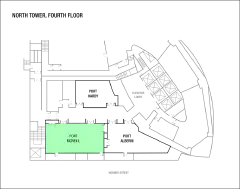## North Tower, 4th floor### 5 December, 2022

##### Abstract

A theorem of Furstenberg from 1967 states that if \Gamma is a lattice in a semisimple Lie group G, then there exists a measure on \Gamma with finite first moment such that the corresponding harmonic measure on the Furstenberg boundary is absolutely continuous. We will discuss generalizations of this theorem in the setting of the Mapping class group and Gromov hyperbolic groups.

##### Abstract

We prove that for each finite index subgroup H of the mapping class group of a closed hyperbolic surface, and for each real number r larger than one, there does not exist a faithful C-r-action (in Hölder’s sense) of H on a circle. For this, we partially determine the critical regularity of faithful actions by right-angled Artin groups on a circle. (Joint with Thomas Koberda and Cristobal Rivas)

##### Abstract

Gromov boundary provides a useful compactification for all infinite-diameter Gromov hyperbolic spaces. It consists of all geodesic rays starting at a given base-point and it has been an essential tool in the study of the coarse geometry of hyperbolic groups. In this study we introduce two topological spaces that are natural analogs of the Gromov boundary for a larger class of metric spaces. First we construct the sublinearly Morse boundaries and show that it is a QI-invariant topological space that can be associated to all finitely generated groups. Furthermore, for many groups, the sublinear boundary can be identified with the Poisson boundaries of the associated group, thus providing a QI-invariant model for Poisson boundaries. This result answers the open problems regarding QI-invariant models of CAT(0) groups and the mapping class group. Lastly, for a subset of the metric spaces we define a compactification of the sublinearly Morse boundary and show that in these cases they are naturally identified with the Bowditch boundary. This talk is based on a series of joint work with Kasra Rafi and Giulio Tiozzo.

##### Abstract

I will report on the joint works with J. Brum, N. Matte-Bon and M. Triestino about R-focal actions of groups on the real line by homeomorphisms. I will describe and motivate this notion, provide some examples and give some applications to the problem of understanding the space of actions of a given (class of) group(s).

### 6 December, 2022

##### Abstract

I will discuss geometric aspects of hyperbolic manifolds related to Dehn filling.

##### Abstract

Among the groups of orientation-preserving homeomorphisms of the circle, the one which preserves a veering pair of laminations are 3-manifold groups. We explain motivation, previous results, and main ingredients of the proof. This is based on the joint work with KyeongRo Kim and Hongtaek Jung.

##### Abstract

We explain how to generalize a classical construction of Thurston for pseudo-Anosov maps to obtain loxodromic elements for the action of a big mapping class group on a ray graph. Our work is based on ideas of Hooper, Thurston and Veech. Joint work with Israel Morales.

##### Abstract

I’ll discuss a proposed notion of geometric finiteness in the mapping class group, motivated by both the analogy with Kleinian groups and the geometry of extension groups, following Farb and Mosher’s work on convex cocompactness. This is joint work with Spencer Dowdall, Matthew Durham, and Alessandro Sisto.

### 8 December, 2022

##### Abstract

Coxeter groups are groups generated by involutions $s_i$, with the relations of form $(s_is_j)^m=$ id. For each Coxeter group, we will be discussing a particular system of “voracious” paths between any two vertices of the Cayley graph. This system turns out to have the “fellow traveller” property and is generated by a finite state automaton. This is joint work with Damian Osajda.

##### Abstract

We show that for every non-elementary hyperbolic group, an associated topological ﬂow space admits a coding based on a transitive subshift of ﬁnite type. Applications include regularity results for Manhattan curves, the uniqueness of measures of maximal Hausdorff dimension with potentials, and the real analyticity of intersection numbers for families of dominated (Anosov) representation, thus providing direct proof of a result established by Bridgeman et al. in 2015. Joint work with Steve Cantrell (Chicago).

##### Abstract

A recent trend in geometric group theory is to understand the large scale geometry of a group with respect to a collection of subgroups. The objects of study are pairs consisting of a finitely generated group and a finite collection of subgroups, and there is a notion of quasi-isometry of pairs inducing an equivalence. This relation captures classical phenomena in the study of quasi-isometric rigidity and brings up natural questions. The talk will introduce some of these topics and discuss recent results obtained in joint work with Sam Hughes and Luis Sánchez Saldaña.